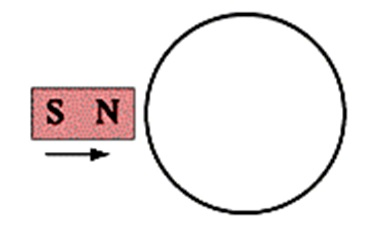# Problem: If a north pole moves toward the loop in the plane of the page, in what direction is the induced current?A. clockwiseB. counterclockwiseC. no induced current

###### FREE Expert Solution

Magnetic flux:

$\overline{){{\mathbf{\varphi }}}_{{\mathbf{B}}}{\mathbf{=}}{\mathbf{B}}{\mathbf{A}}{\mathbf{c}}{\mathbf{o}}{\mathbf{s}}{\mathbf{\theta }}}$ where θ is the angle between B and A.

In this problem, we are going to apply Lenz's law to get the direction of the induced current.

Lenz's law: The induced electric current flows in a direction such that the current opposes the change in magnetic flux inducing it.

87% (479 ratings)###### Problem Details

If a north pole moves toward the loop in the plane of the page, in what direction is the induced current?A. clockwise

B. counterclockwise

C. no induced current